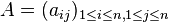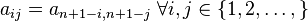# Centrosymmetric matrix

This article defines a property that can be evaluated for a square matrix. In other words, given a square matrix (a matrix with an equal number of rows and columns) the matrix either satisfies or does not satisfy the property.
View other properties of square matrices

## Definition

### Verbal definition

A centrosymmetric matrix is a square matrix that commutes with the exchange matrix (the matrix with 1s on the antidiagonal and 0s elsewhere) of the same number of rows and columns.

### Algebraic definition

Supposeis a positive integer. Amatrixis termed a centrosymmetric matrix if it satisfies the following condition:In symbols, ifdenotes the exchange matrix, thenis centrosymmetric if and only if.

## Relation with other properties

### Stronger properties

Property Meaning Proof of implication Proof of strictness (reverse implication failure) Intermediate notions